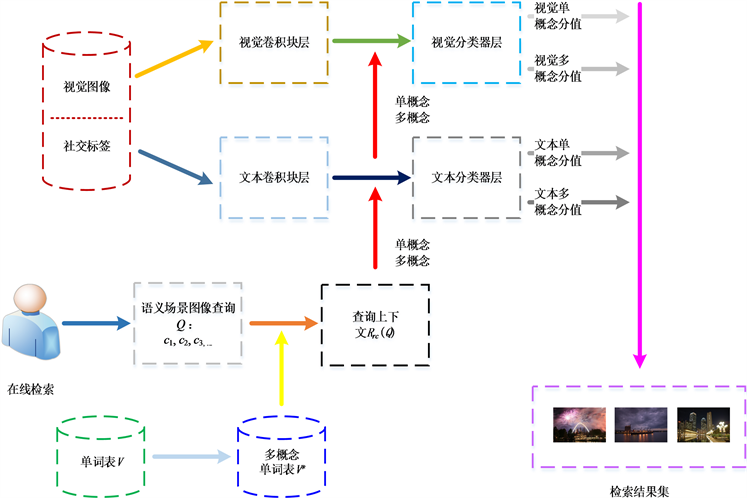# 基于深度学习的语义场景图像检索Deep Learning Based Semantic Scene Image Retrieval

DOI: 10.12677/CSA.2019.98175, PDF, HTML, XML, 下载: 373  浏览: 824  科研立项经费支持

Abstract: With the explosive growth of multimedia objects such as Web images over the Internet, online semantic scene image retrieval has been receiving increasing research interest. Conventional studies focus on single-concept-based image retrieval and cannot effectively retrieve semantic scene images including multiple concepts that describe characteristic semantic scene. To tackle this issue, i.e., semantic scene Web image retrieval, we propose a novel approach called multi-modal deep learning based Semantic Scene Image Retrieval (SSIR) in this paper. In particular, we first train a multi-modal Convolutional Neural Network (CNN) as a concept classifier for images and texts. Second, semantic interdependencies of the subconcepts included in the images are utilized to refine the predicted semantic scores in order to enhance holistic scene recognition. Finally, to improve the performance of retrieving rare scene concepts, a gradient descent algorithm is used for compensating the varying frequencies of concepts derived from imbalanced image datasets. The results of our experiments on MIR Flickr 2011 have shown that our proposed approach performs favorably compared with several traditional methods.

1. 引言Figure 1. The graphical illustration of semantic scene image retrieval

2. 基于多模态深度学习的语义场景图像检索

2.1. 问题描述

2.2. 系统框架

SSIR检索框架如图2所示，包含3个部分：卷积块层、分类器层、在线检索。卷积块层学习出两类特征：深度视觉特征与深度文本特征。通过视觉卷积块层，将图像像素转换为视觉特征向量；通过文本卷积块层，将关联的文本社交标签转换为词嵌入  ，然后学习出文本特征向量。分类器层学习出从特征到概念的映射函数，包含两种分类器：单概念目标分类器和多概念场景分类器。这里，需要生成多概念单词表V*。由于V*中的概念分为语义单概念和场景多概念两类，所以共有四类映射函数：单概念视觉映射函数、多概念视觉映射函数、单概念文本映射函数、多概念文本映射函数。在线检索根据给定的多概念语义场景查询Q从测试集中检索到最相关的图像结果。由于要使用多概念之间的语义依赖关系，因此需要生成查询上下文Rrc(Q)。Figure 2. The SSIR system framework

$|{C}_{i}|\le t$ (1)

$|{N}_{r}\left({c}_{1},\cdots ,{c}_{k}\right)|\ge s$ (2)

2.3. 多模态CNN结构

$p\left({C}_{j}|I\right)=\frac{\mathrm{exp}\left({q}_{j}\left(I\right)\right)}{{\sum }_{k}\mathrm{exp}\left({q}_{k}\left(I\right)\right)},$ (3)

${f}_{\text{softmax}}\text{}=-\frac{1}{N}{\sum }_{i}{\sum }_{j}{\stackrel{¯}{p}}_{i,j}\mathrm{log}\left(p\left({C}_{j}|I\right)\right),$ (4)

2.4. 语义场景查询上下文Rrc(Q)的生成

$p\left(Q|{C}_{i}\right)=\frac{2×Nr\left(Q,{C}_{i}\right)}{Nr\left(Q\right)+Nr\left({C}_{i}\right)}$ (5)

$p\left(Q|{C}_{i}\right)=\left\{\begin{array}{l}\frac{p\left(Q|{C}_{i}\right)}{\underset{r=1}{\overset{{K}_{rc}}{\sum }}p\left(Q|{C}_{r}\right)}\text{if}{C}_{i},{C}_{r}\in {R}_{rc}\left(Q\right)\\ 0\text{elsewhere}\text{.}\end{array}$ (6)

2.5. 多模态场景语义映射

$r\left(Q,\left(I,T\right)\right)=r\left(Q,I\right)+r\left(Q,T\right)$ (7)

$r\left(Q,I\right)={\alpha }_{1}×{r}_{s}\left(Q,I\right)+{\alpha }_{2}×{r}_{m}\left(Q,I\right)$ (8)

$r\left(Q,T\right)={\alpha }_{3}×{r}_{s}\left(Q,T\right)+{\alpha }_{4}×{r}_{m}\left(Q,T\right)$ (9)

${r}_{s}\left(Q,I\right)=\underset{i=1}{\overset{t}{\prod }}p\left({c}_{i}|I\right),$ (10)

${r}_{m}\left(Q,I\right)=\underset{i=1}{\overset{{K}_{rc}}{\sum }}p\left(Q|{C}_{r}\right)×p\left({C}_{r}|I\right),\text{\hspace{0.17em}}\text{\hspace{0.17em}}{C}_{r}\in {R}_{rc}\left(Q\right),$ (11)

${r}_{s}\left(Q,T\right)=\underset{i=1}{\overset{t}{\prod }}p\left({c}_{i}|T\right),$ (12)

${r}_{m}\left(Q,T\right)=\underset{i=1}{\overset{{K}_{rc}}{\sum }}p\left(Q|{C}_{r}\right)×p\left({C}_{r}|T\right),\text{\hspace{0.17em}}\text{\hspace{0.17em}}{C}_{r}\in {R}_{rc}\left(Q\right),$ (13)

2.6. SSIR参数估算

$p\left({y}_{{Q}_{i}}=1\right)=r\left(Q,\left(I,T\right)\right),$ (14)

$p\left({y}_{{Q}_{i}}=0\right)=1-r\left(Q,\left(I,T\right)\right),$ (15)

$p\left({y}_{{Q}_{i}}\right)=\text{}p{\left({y}_{{Q}_{i}}=1\right)}^{{y}_{{Q}_{i}}}p{\left({y}_{{Q}_{i}}=0\right)}^{1-{y}_{{Q}_{i}}}$ (16)

${L}_{Q}=\underset{i=1}{\overset{N}{\sum }}{n}_{{Q}_{i}}\mathrm{log}p\left({y}_{{Q}_{i}}\right)$ (17)

${L}_{Q}=\underset{i=1}{\overset{N}{\sum }}{n}_{{Q}_{i}}\mathrm{log}\left\{{\left(\alpha \beta \right)}^{{y}_{{Q}_{i}}}{\left(1-\alpha \beta \right)}^{1-{y}_{{Q}_{i}}}\right\}$ (18)

$\beta =\left\{\underset{i=1}{\overset{t}{\prod }}p\left({c}_{i}|I\right),\underset{i=1}{\overset{{K}_{rc}}{\sum }}p\left(Q|{C}_{r}\right)×p\left({C}_{r}|I\right),\underset{i=1}{\overset{t}{\prod }}p\left({c}_{i}|T\right),\underset{i=1}{\overset{{K}_{rc}}{\sum }}p\left(Q|{C}_{r}\right)×p\left({C}_{r}|T\right)\right\}.$

3. 实验和评价

3.1. 数据集

3.2. 实验结果与分析Table 1. Performance comparisons of semantic scene image retrieval

2019年广东第二师范学院校级教学质量与教学改革工程项目(编号：2019jxgg18)；广东第二师范学院软件工程重点学科建设项目(编号：9030-1700207)；广东省自然科学基金项目(编号：2018A0303130169)；广东省科技计划项目(编号：粤财农94号，2016A010106007)；广东省应用型科技研发专项资金资助项目(编号：2016B090927010)；2019年广东第二师范学院大学生创新创业训练计划项目(编号：201914278146)。

  熊回香, 叶佳鑫. 基于同义词词林的社会化标签等级结构构建研究[J]. 情报杂志, 2018, 37(1): 126-131.  龚彦宇, 董剑利, 侯明亮. 综合颜色特征的图像检索方法研究[J]. 计算机科学与应用, 2016, 6(10): 583-589.  Fang, Q., Xu, C. and Sang, J. (2016) Folksonomy-Based Visual Ontology Construction and Its Applica-tions. IEEE Transactions Multimedia, 18, 702-713. https://doi.org/10.1109/TMM.2016.2527602  Wang, W., Yang, X. and Ooi, B.C. (2016) Effective Deep Learning Based Multi-Modal Retrieval. The VLDB Journal, 25, 79-101. https://doi.org/10.1007/s00778-015-0391-4  杨泽明, 刘军, 薛程, 于子红. 卷积神经网络在图像分类上的应用综述[J]. 人工智能与机器人研究, 2018, 7(1): 17-24.  叶天顺. 一种改进的社交词嵌入算法[J]. 计算机应用与软件, 2018(9): 132-137.  He, K., Zhang, X., Ren, S. and Sun, J. (2016) Deep Residual Learning for Image Recognition. IEEE Conference on Computer Vision and Pattern Recognition, Las Vegas, 27-30 June 2016, 770-778. https://doi.org/10.1109/CVPR.2016.90  Kim, Y. (2014) Convolutional Neural Networks for Sentence Classi-fication. ACL International Conference on Empirical Methods in Natural Language Processing, Doha, 25-29 October 2014, 1746-1751. https://doi.org/10.3115/v1/D14-1181  陶秉墨, 鲁淑霞. 基于自适应随机梯度下降方法的非平衡数据分类[J]. 计算机科学, 2018, 45(z1): 487-492.  Nowak, S., Nagel, K. and Liebetrau, J. (2011) The CLEF 2011 Photo Annotation and Concept-Based Retrieval Tasks. CLEF Conference and Labs of the Evaluation Forum, Amsterdam, 19-22 September 2011, 1-25.  李鹏, 蒋品群, 曾上游, 夏海英, 廖志贤, 范瑞. 基于分组残差结构的轻量级卷积神经网络设计[J]. 微电子学与计算机, 2019, 36(7): 43-47.  王雅慧, 刘博, 袁晓彤. 基于近似牛顿法的分布式卷积神经网络训练[J]. 计算机科学, 2019, 46(7): 180-185.  张涛, 杨剑, 宋文爱, 郭雁蓉. 改进卷积神经网络模型设计方法[J]. 计算机工程与设计, 2019, 40(7): 1885-1890.  程俊华, 曾国辉, 鲁敦科, 黄勃. 基于Dropout的改进卷积神经网络模型平均方法[J]. 计算机应用, 2019, 39(6): 1601-1606.  Simonyan, K. and Zis-serman, A. (2014) Very Deep Convolutional Networks for Large-Scale Image Recognition.  Guillaumin, M., Ver-beek, J.J. and Schmid, C. (2010) Multimodal Semi-Supervised Learning for Image Classification. The 23rd IEEE Con-ference on Computer Vision and Pattern Recognition, San Francisco, 13-18 June 2010, 4307-4311. https://doi.org/10.1109/CVPR.2010.5540120  Srivastava, N. and Salakhutdinov, R. (2014) Multimodal Learn-ing with Deep Boltzmann Machines. Journal of Machine Learning Research, 15, 2949-2980. https://doi.org/10.1162/NECO_a_00311